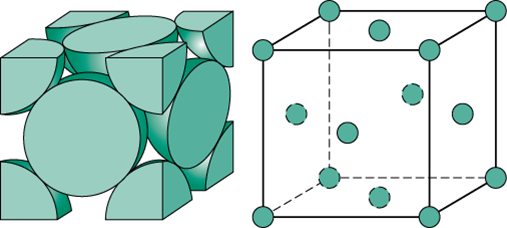# An element has a face-centred cubic (fcc) structure with a cell edge of a. The distance between the centres of two nearest tetrahedral voids in the lattice is :Option 1)Option 2)aOption 3)Option 4)

No. of atoms(z) for face centered unit cell -

Lattice points: at corners and face centers of unit cell.

For face centered cubic (FCC), z=4.

- whereinRelation between radius of constituent particle, r and edge length, a for face centered cubic unit cell --

We know that, in fcc cell unit, the tetrahedral voids are located at body diagonal at a distance  from the corners.

Now,(Tv= tetrahedral voids)

Now,  &  are similar triangle,

distance between two nearest tetrahedral voids is

Option 1)

Option 2)

a

Option 3)

Option 4)

Exams
Articles
Questions